Physical Chemistry : Michaelis-Menton Analysis

Example Questions

Example Question #7 : Reaction Kinetics

Which of the following is true regarding the Michaelis constant?

It is the enzyme concentration at which the reaction rate is half of maximum

It is the enzyme concentration at which the reaction rate is at maximum

It is the substrate concentration at which the reaction rate is at maximum

It is the substrate concentration at which the reaction rate is half of maximum

It is the substrate concentration at which the reaction rate is half of maximum

Explanation:

Michaelis constant, or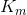, is defined as the concentration of substrate at which the reaction rate is half the maximum (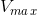). It is a useful measure of how much substrate is needed for reaction to proceed rapidly. A reaction with a high Michaelis constant will need lots of substrate to reach high reaction rates whereas a reaction with low Michaelis constant will need small amounts of substrate to reach high reaction rates.

Example Question #8 : Reaction Kinetics

Which of the following will have the greatest increase in reaction rate?

Decreasing the substrate concentration by a factor of 2

Increasing the Michaelis constant by a factor of 2

Increasing the maximum velocity by a factor of 2

Increasing the substrate concentration by a factor of 2

Increasing the maximum velocity by a factor of 2

Explanation:

Reaction rate, according to Michaelis-Menten model is as follows.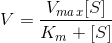whereis reaction rate,is maximum reaction rate,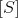is substrate concentration, andis the Michaelis constant. If we analyze the given options, we will observe that the greatest increase inoccurs whenis doubled (increased by a factor of 2). Increasing substrate concentration by a factor of 2 will have nearly the same effect; however, sinceis also found in the denominator it will only slightly contribute to an increase in.

Note that the units foris molarity,is molarity, andis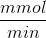. Solving forwill give us units of.

Example Question #1 : Michaelis Menton Analysis

Consider the following reaction parameters.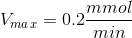Substrate concentration =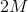Michaelis constant =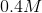What can you conclude about the reaction rate?

The reaction rate is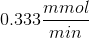The reaction rate is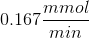The reaction rate is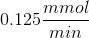The reaction rate cannot be determined from the given information

The reaction rate isExplanation:

To solve this problem we need to use the Michaelis-Menten equation.whereis reaction rate,is maximum reaction rate,is substrate concentration, andis the Michaelis constant. If we plug in the given values we get a reaction rate of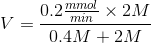Note that the Michaelis-Menten equation implies that thewill never exceed. Regardless of how high the substrate concentration is, the reaction rate will approachbut will never equal or exceed it. You can try this by substituting very high values for substrate concentration. Thewill get very close to 0.2 () but will never equal or exceed it.

Example Question #10 : Reaction Kinetics

The Michaelis-Menten model implies that __________ the Michaelis constant will __________ the reaction rate.

increasing . . . not change

decreasing . . . not change

decreasing . . . decrease

increasing . . . decrease

increasing . . . decrease

Explanation:

The Michaelis-Menten equation is as follows.Whereis reaction rate,is maximum reaction rate,is substrate concentration, andis the Michaelis constant. Since the Michaelis constant,, is in the denominator, the reaction rate is inversely proportional to the Michaelis constant; therefore, increasing the Michaelis constant will decrease the reaction rate.

All Physical Chemistry Resources